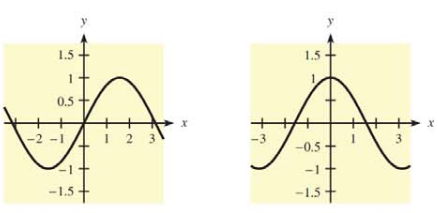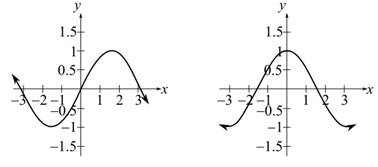Chapter 10.1, Problem 47E### Mathematical Applications for the ...

11th Edition
Ronald J. Harshbarger + 1 other
ISBN: 9781305108042

#### Solutions

Chapter
Section### Mathematical Applications for the ...

11th Edition
Ronald J. Harshbarger + 1 other
ISBN: 9781305108042
Textbook Problem

# In Problems 47 and 48, two graphs are given. One is the graph of f , and the other is the graph of f Decide which is which and explain your reasoning.To determine

The graph of the function f(x) and the graph of the derivative f'(x) which are the two graphs are given and explain the answer.Explanation

Given Information:

The provided graphs of f(x) and f(x) are as follows:

Explanation:

Consider the provided graphs,

For function f which is differentiable in interval (a,b),

If f(x)>0 then function f is increasing in that interval and if f(x)<0 then function f is decreasing in that interval

### Still sussing out bartleby?

Check out a sample textbook solution.

See a sample solution

#### The Solution to Your Study Problems

Bartleby provides explanations to thousands of textbook problems written by our experts, many with advanced degrees!

Get Started

#### Prove the identity. 52. 11sin+11+sin=2sec2

Single Variable Calculus: Early Transcendentals, Volume I

#### (2, 5]

Applied Calculus for the Managerial, Life, and Social Sciences: A Brief Approach

#### In Exercises 1-6, simplify the expression. 3+2x7x

Calculus: An Applied Approach (MindTap Course List)

#### Find the value of the sum. 32. i=1n(3+2i)2

Single Variable Calculus: Early Transcendentals

#### Which curve is simple but not closed?

Study Guide for Stewart's Multivariable Calculus, 8th

#### Sometimes, Always, or Never: If limxaf(x) and f(a) both exist, then f is continuous at a.

Study Guide for Stewart's Single Variable Calculus: Early Transcendentals, 8th